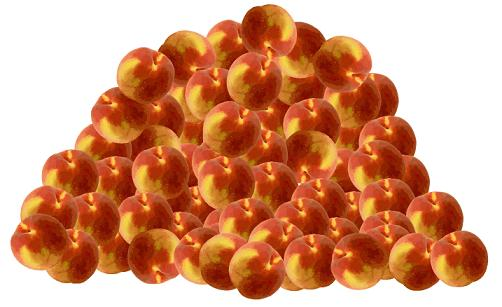#### You may also like### Triangle Incircle Iteration

Keep constructing triangles in the incircle of the previous triangle. What happens?### Loopy

Investigate sequences given by $a_n = \frac{1+a_{n-1}}{a_{n-2}}$ for different choices of the first two terms. Make a conjecture about the behaviour of these sequences. Can you prove your conjecture?### Converging Means

Take any two positive numbers. Calculate the arithmetic and geometric means. Repeat the calculations to generate a sequence of arithmetic means and geometric means. Make a note of what happens to the two sequences.

# Peaches in General

##### Age 14 to 16 Challenge Level:There's a problem which goes like this :

He ate half of them plus one more.

On the second day, he ate half of the rest plus one more.

On the third day, he ate half of the rest plus one more again.

On the fourth day, he found there was only one left.

How many did he have at the beginning?

By the time you reach Stage 4 you will start to feel that problems like this are examples or instances of something more general.

Try this problem as it is and then generalise your solution as far as you think you can.

Using a spreadsheet may be a help, but you can judge that for yourself.